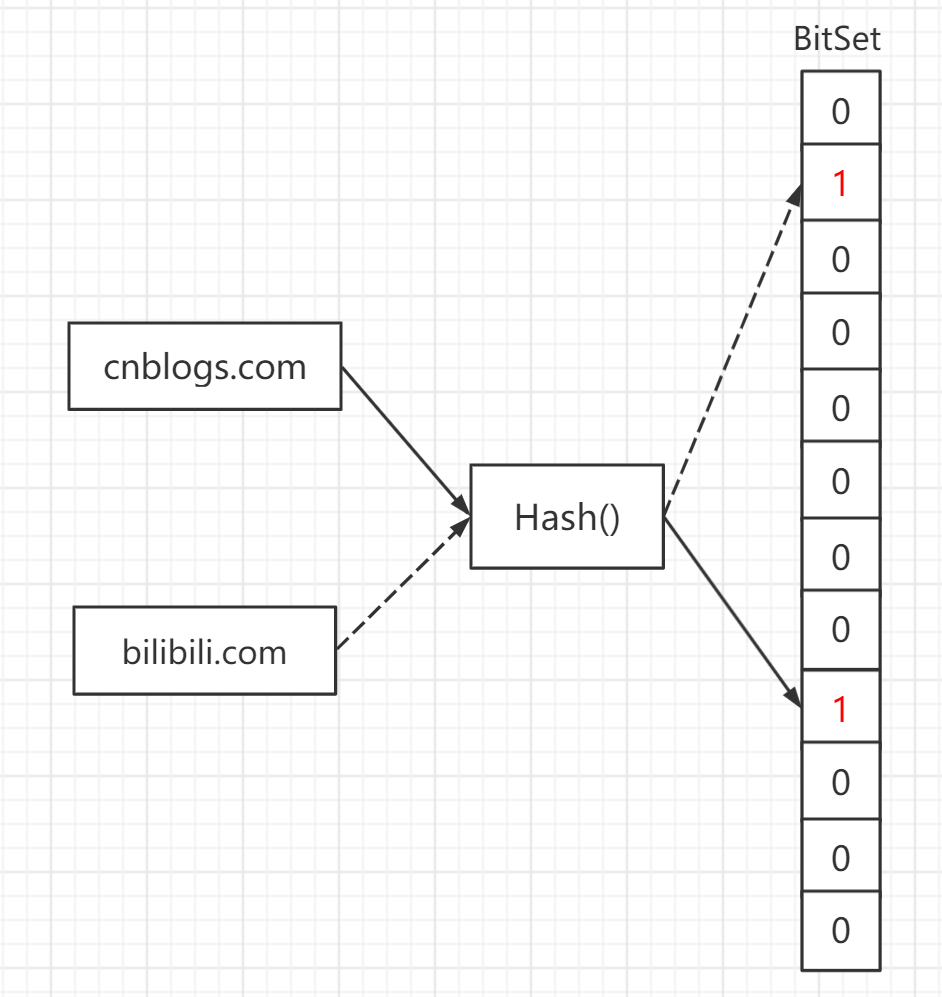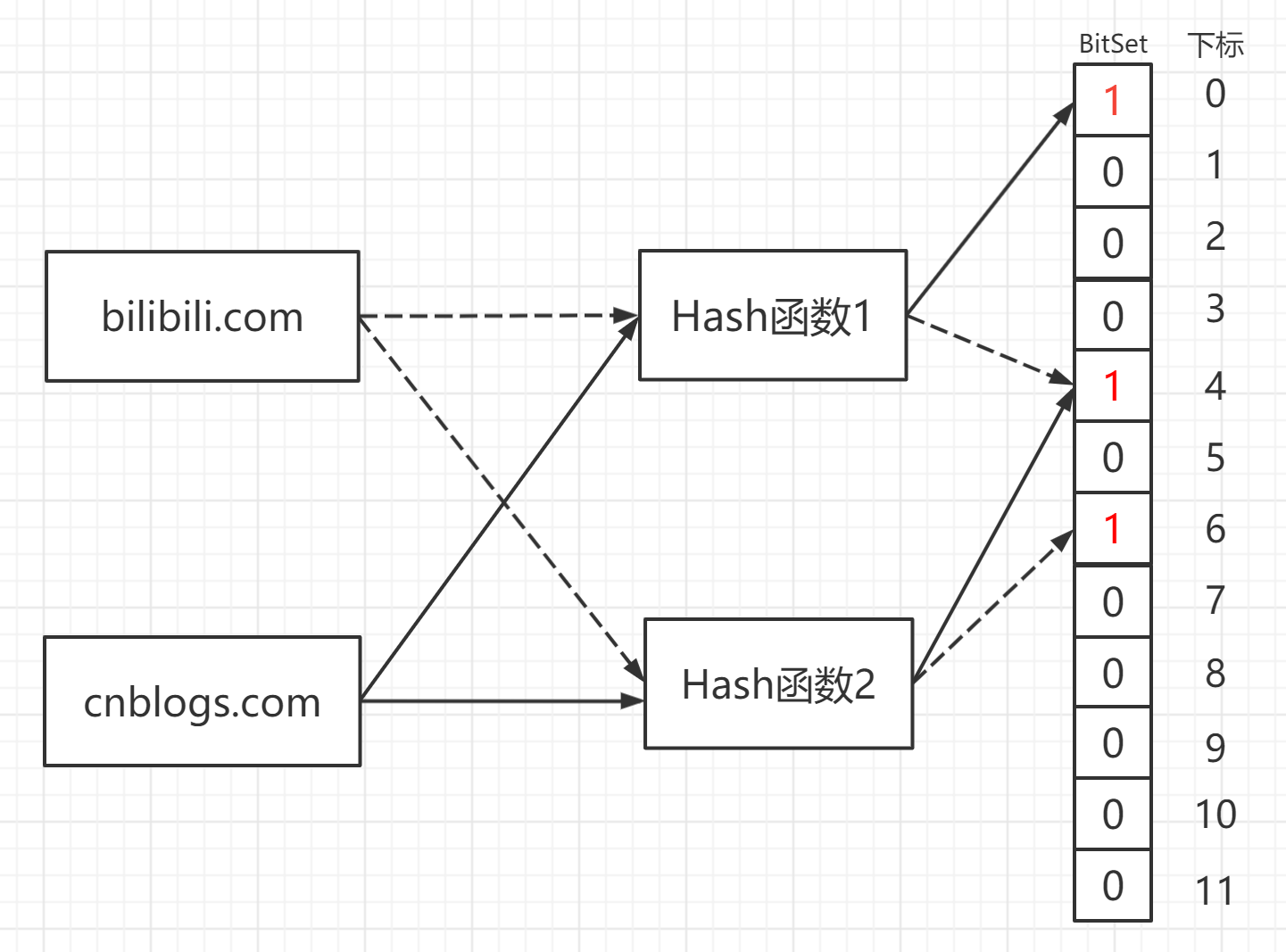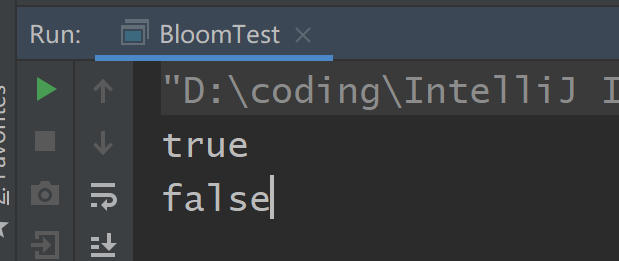# 布隆过滤器（Bloom Filter）详解及应用

## 1 位图（BitMap）## 2 布隆过滤器（Bloom Filter）

### 2.1 是什么$Hash_1(bilibli.com) \% 12 = 4$

$Hash_2(bilibli.com) \% 12 = 6$

### 2.2 误判率

$\frac1m$

$1-\frac1m$

$(1-\frac1m)^k$

$\lim\limits_{m\rightarrow\infty}(1-\frac1m)^m=\frac1e$

$(1-\frac1m)^k\approx e^{\frac{-k}{m}}$

$(1-\frac1m)^{kn}\approx e^{\frac{-kn}{m}}$

$1-e^{\frac{-kn}{m}}$

$P\approx (1-e^{\frac{-kn}{m}})^k$

P即为布隆过滤器，将n条数据，进行k次哈希后，存入大小为m的bit数组后，再查询一条数据，出现误判（数据不存在，却误以为存在）的概率。### 2.3 使用（以Java为例）

<dependency>
<artifactId>guava</artifactId>
<version>28.0-jre</version>
</dependency>


BloomFilter<String> filter = BloomFilter.create(
Funnels.stringFunnel(Charset.defaultCharset()),
1000,
0.001);


filter.put("bilibili.com");
filter.put("cnblogs.com");

System.out.println(filter.mightContain("bilibili.com"));
System.out.println(filter.mightContain("zhihu.com"));### 2.4 实际应用

• 识别恶意邮箱地址
• URL黑名单、白名单，比如Chrome浏览器就是使用了一个布隆过滤器识别恶意链接。
• 解决缓存穿透问题，缓存穿透指查询一个不存在的数据，这时候缓存中不存在，就会不断的查询数据库，造成不必要的IO，而且有人如果恶意使用不存在的key也可以对数据库进行攻击。
• 果蝇....通过改进的布隆过滤器来检测新鲜气味。（混入一个奇怪的东西）
• 谷歌Bigtable、Apache HBase、Apache Cassandra和PostgreSQL使用布隆过滤器来减少对不存在的行或列的磁盘查找
• ......

## 3 布谷鸟过滤器

https://www.cnblogs.com/zhaodongge/p/15067657.html

posted @ 2021-07-15 21:20  泰阁尔  阅读(3498)  评论(3编辑  收藏  举报